# A “top-down” approach for volume data

Ashwood, Melbourne, Australia

(posted April 23, 2010; revised January 18, 2011)

Abstract

I propose that volume data should be stored, retrieved, processed, and visualized in a “top-down” manner, using pyramid encoding (mipmapping), exploiting the remarkable properties of the “magic kernel” resampling method. The same kernel can also be used to upsample and downsample in the temporal direction for time-varying datasets.

## Introduction

Most volume data is handled in a “bottom-up” manner, namely, the voxel data at the full captured or computed resolution is considered to be the primary data, and multi-resolution pyramids—where they are used at all—are generally derived from this primary data in an extra processing step, for the purposes of more rapid visualization through progressive refinement.

In this paper I argue that top-down pyramid encoding (mipmapping) is practicable for volume data, using the “magic kernel” for downsampling and upsampling any image in any direction by a factor of two. This resampling is lightning-fast, yet yields resampled images that are astoundingly free of aliasing artifacts.

In recent years I have successfully applied this “top-down” philosophy to two-dimensional images (see here). In this paper I show its generalization to three-dimensional volume data.

## The magic kernel on volume data

In the following example I have made use of the Drishti volume rendering application, and a sample scan of a stag beetle, 494 x 832 x 832 voxels of byte-valued data (326 MiB uncompressed, or 12.6 MiB when compressed with gzip):Downsampling this volume data once (by a factor of two in each direction) using the magic kernel yields the following 247 x 416 x 416 representation (40.8 MiB uncompressed, 1.8 MiB compressed):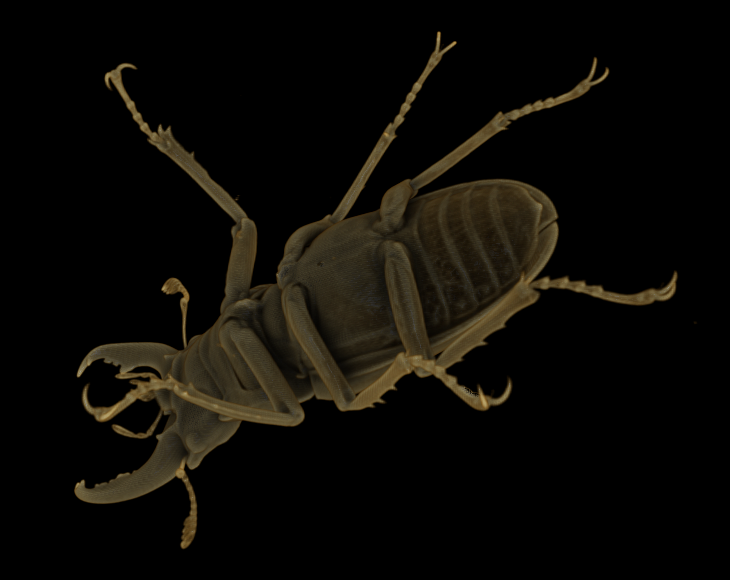Downsampling again yields the following 124 x 208 x 208 (note that dimensions are rounded up when they are halved) representation (5.2 MiB uncompressed, 267 KiB compressed):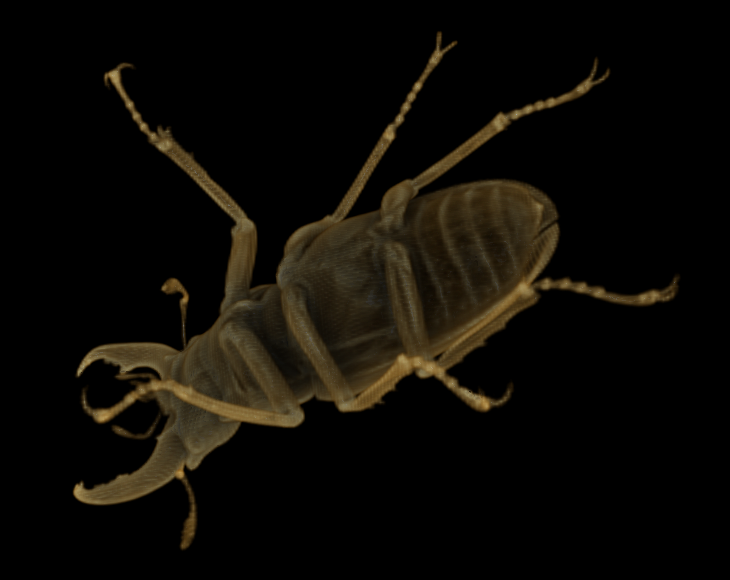Despite only having 1/64 of the data of the original representation (or 1/48 in compressed form), this representation is still useful. Downsampling again yields the following 62 x 104 x 104 representation (655 KiB uncompressed, 42 KiB compressed):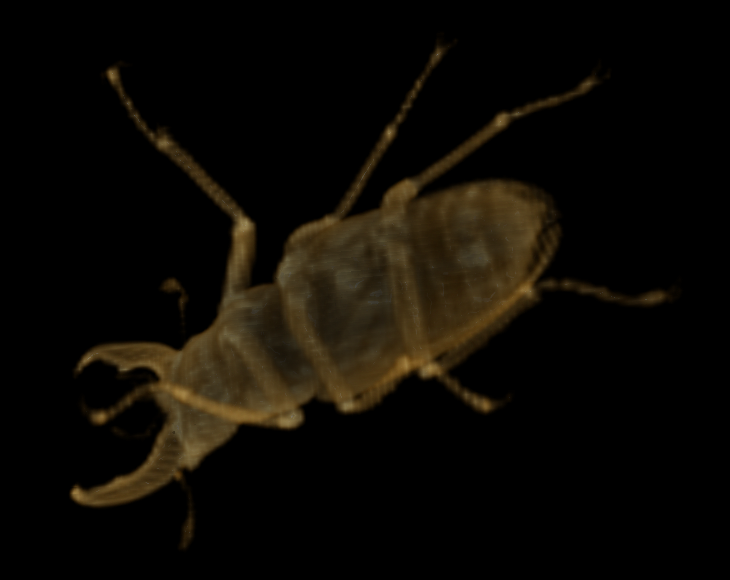At only 1/512 of the data of the original representation (1/315 compressed), this approximation is arguably still useful. Downsampling again yields the following 31 x 52 x 52 representation (82 KiB uncompressed, 7.4 KiB compressed):This is arguably useful as a low-resolution representation. A further downsampling, to the following 16 x 26 x 26 representation (11 KiB uncompressed, 1.6 KiB compressed), pushes the usefulness of the downsampled representation to its practical limit: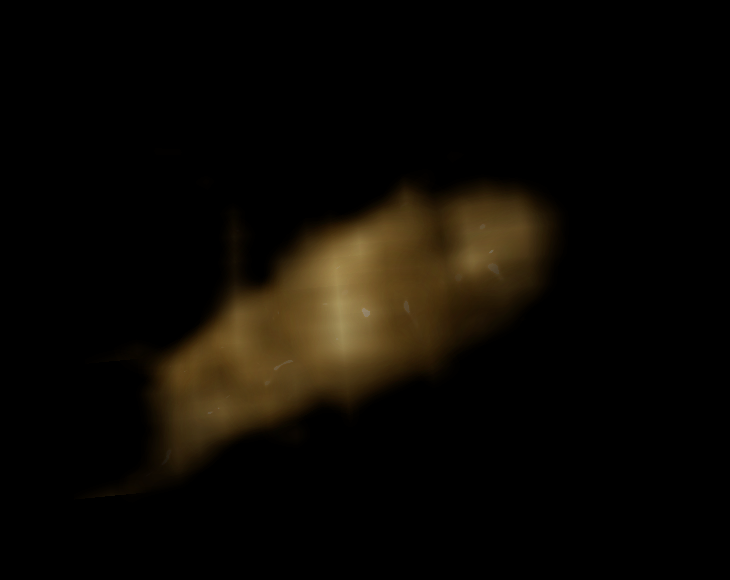(Interestingly, the same scale of dimensions proves to be the minimum useful representation in the two-dimensional case: see here). Pushing it one more level, to the following 8 x 13 x 13 representation (1.4 KiB uncompressed, 381 bytes compressed), yields an object too amorphous for the renderer to generate any useful visual representation (arguably a reasonable limitation, for only 381 bytes of actual information):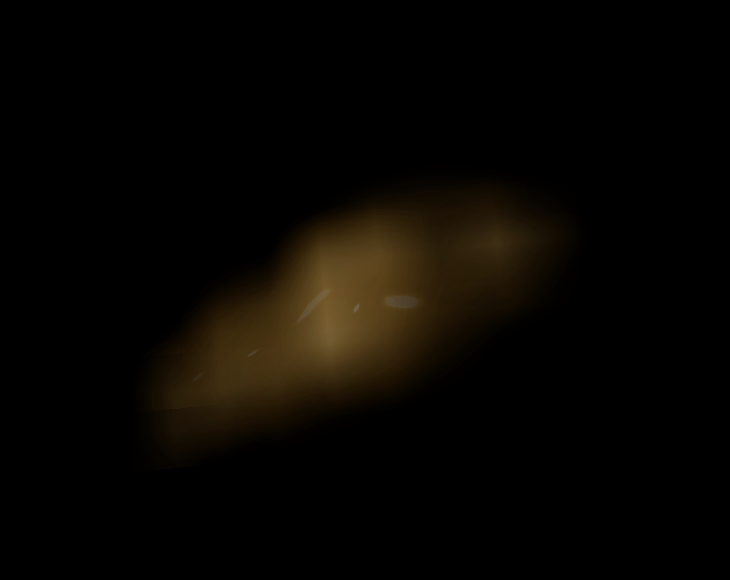## Top-down storage paradigm

The example above suggests that it may be useful for a visualization tool to generate the lower-resolution magic kernel representations of an object after loading in the full-resolution dataset. However, pyramid encoding (mipmapping) allows us to turn this multi-resolution pyramid upside-down (or right side up, one could argue): a visualization tool can not only get these approximations essentially for free, but moreover can access them without needing to access the full-resolution dataset at all.

Although not useful for visualization purposes, consider a continuation of the downsampling process for the example shown in the previous section: we successively get a 4 x 7 x 7 representation (196 bytes), a 2 x 4 x 4 representation (32 bytes), a 1 x 2 x 2 representation (4 bytes), and finally a 1 x 1 x 1 representation (1 byte).

I shall refer to this ultimate, single-voxel representation—inside which the entire beetle is contained—as the fundamental voxel, and also as the scale-0 representation. It is essentially the spatial average value of the original dataset.Let us now (conceptually, at least) store this scale-0 representation in its own data file. The data we store will in general only be an approximation, due to round-off in each downsampling (which depends on the number of bits per voxel we use for each successive representation, which need not be the same at each scale; in the above example, I have stored each representation in byte-valued arrays); and we might also, in general, choose to lossily encode the data before storing it, to save space. I shall refer to what is stored as the scale-0 approximation.

We now use the magic kernel to upsample the scale-0 approximation to 1 x 2 x 2 voxels, which I shall refer to as the upsampled scale-0 approximation. We now compute the difference between the scale-1 representation and the upsampled scale-0 approximation, which I shall refer to as the scale-1 diff. We now store the scale-1 diff in its own file.

Again, this diff might, in general, be lossily encoded. We therefore reload and decode the scale-1 diff, and add the result to the upsampled scale-0 approximation, to yield the scale-1 approximation. This is the best approximation that can be reconstructed, at scale 1, using only the two data files that we have stored.

We now iterate this process until we get up to the resolution of the original representation (which, for this example, is scale 10). At each scale, we store only the diff file. (The scale-0 approximation can be also be considered a “diff” from an initial approximation of zero.)

I propose that this set of diff files be used as a complete replacement for the original full-resolution dataset. (If the original dataset must be able to be retrieved losslessly, but lossy encoding has been used for the diffs, then the storage of an additional, losslessly-encoded “final diff”, between the final-scale representation and the final-scale approximation, will suffice.)

In practice, of course, the minimum scale stored would not be scale 0, but rather something more closely aligned with the minimum file size that can be efficiently stored on the file system used (say, scale 5).

From an information theory perspective, it is clear that the Shannon information contained in the set of diffs is equal to that contained in the original dataset; thus, if we are clever enough in our encoding, the amount of storage required for the diff file set should be roughly the same as would be required for the original dataset.

For example, considered in Fourier space, the lower half of the Fourier spectrum (in each direction) of the scale-n representation should be simply the Fourier spectrum of the scale-(n - 1) representation (as it has simply been resampled onto a new lattice with half the lattice spacing), so that the lower half of the Fourier spectrum of the scale-n diff should be zero. (In practice, edge effects, and the difference between the magic kernel and the sinc function, means that the lower half of the spectrum will be only approximately zero, but this viewpoint is still illustrative.)

This general property also strongly suggests that encodings exploiting Fourier space—whether lossy or lossless—are likely to be the simplest to implement (at the possible expense of decoding speed). But even a naive implementation in position-space can get sufficiently close to the theoretical limit for practical purposes: such a (lossless) implementation for the stag beetle example above yields a gzipped diff file set of 15.0 MiB, compared to the 12.6 MiB of the original dataset.

It is also evident that the vast majority of the data size comes from the final diff. Now, since the lower diffs are already providing such an excellent approximation (thanks to the remarkable properties of the magic kernel), a practical implementation may choose to encode the final diff far more lossily, if storage space is at a premium, to get the best trade-off between representational fidelity and storage requirements.

Now, clearly, a visualization tool only needs to read in the diff files up to the scale it wishes to render, making progressive refinement lightning-fast: a “thumbnail” can be loaded and rendered essentially instantaneously, regardless of how large the final-scale dataset for that particular object happens to be. Indeed, diff files for different scales can be stored in different directories, or even on physically different storage devices or networks. Extra scales can be loaded in, behind the scenes, when the rendering load is light, and dropped again when the rendering load becomes heavy.

The top-down approach also lends itself to a more efficient storage paradigm for extremely large datasets. Imagine that our original dataset is 100,000 x 100,000 x 100,000 voxels. For most visualization purposes, it will be rare to require all of the quadrillion voxels at the same time: a 1024 x 1024 x 1024 subvolume (or 2048, or 4096, say: pick your favorite number) is likely to be sufficient to exhaust most display resolution requirements. Thus, when visualizing the entire object, it should be rare to need more than scales 0 through 10 (or 11 or 12). Higher scales would only be needed when zooming in on a subvolume.

With this in mind, it makes sense to partition each higher-scale dataset into 1024 x 1024 x 1024 subcubes, and store the scale-n diff for each subcube in its own file. The visualization tool will then never need to load up the files for more than eight such subcubes. The top-down paradigm makes such a “gigavoxel file” model possible at every scale—regardless of whether the entire dataset contains a quadrillion voxels, or indeed any higher power of ten.

In the Appendix I define the concept of top-down coordinates, which provides conceptual and technical assistance in writing the compute code for view volumes at any scale using the top-down storage paradigm.

## Visualizing diffs directly

A side-benefit of using the magic kernel is that the set of diffs usefully segments the information in the original volume data into the information at each scale. Visualizing each separate diff may therefore be of use in applications such as medical imaging.

As a naive example, if we simply render the volume obtained from storing the absolute value of each diff value, then at scale 7 we find the following:At scale 8 we find the following: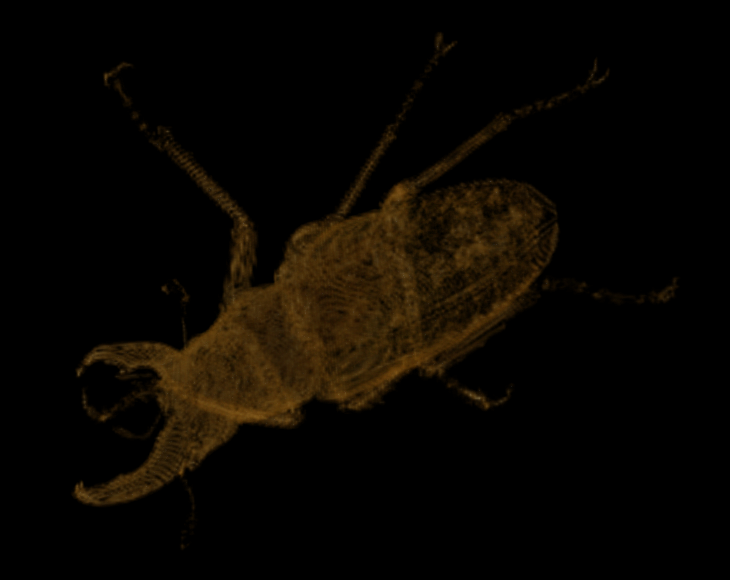At scale 9 we find the following: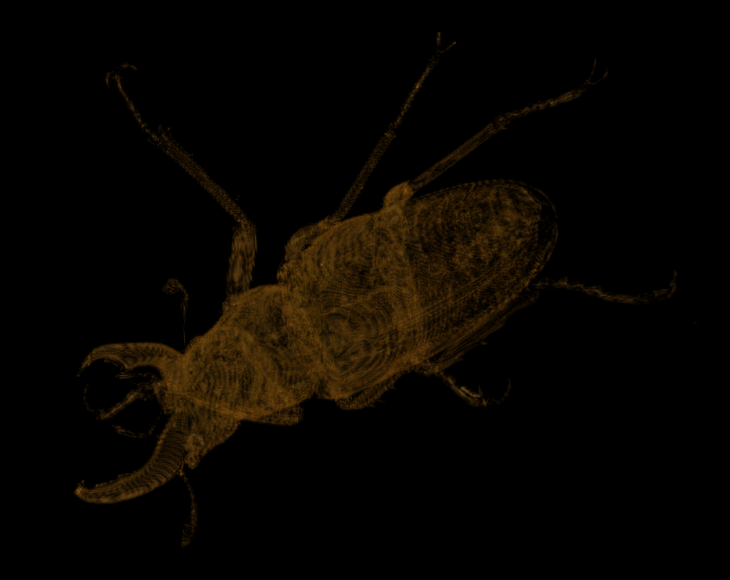And at the original scale, scale 10 we find the following:This is an extremely efficient way of visualizing the interior of objects at different scales, which we get for free when we use the magic kernel.

## The magic kernel in the rendering engine

To this point, we have only considered the use of the magic kernel in the representation of the volume data. However, the same philosophy may be fruitfully employed in all aspects of the visualization pipeline.

For example, if only a low-resolution representation of an object has been loaded from storage, then an equivalently low-resolution rendering engine can be launched, to yield a similarly low-resolution rendered two-dimensional image. This low-resolution image can then up upsampled using the magic kernel, yielding an optimally upsampled image to match the display resolution.

Because three-dimensional rendering engines are not, in general, linear operations, upsampling the rendered image will not, in general, be equivalent to rendering the upsampled volume data: these two operations will not, in general, commute. However, they may be sufficiently commutative for practical use, or the alternatives may be useful for different purposes.

## The magic kernel in the temporal direction for time-varying volume data

In the (3+1)-dimensional case of time-varying volume data, the magic kernel can be fruitfully used to upsample and downsample in the temporal direction. However, the typical uses of temporal upsampling and downsampling are fundamentally different to those of the spatial directions, and so it is important to not try to treat such datasets as simply having four spatial dimensions, but to rather keep the time direction completely isolated from the spatial directions. Simply put: store the volume data at each time step as per the above description; do not (in general) downsample in the time direction before storing the data.

Temporal upsampling can be used to provide a “slow motion” view that does not “jump” from frame to frame. Slowing down time by a factor of 1/2 can be obtained by simply piping the data through the upsampling filter, which only requires two “frames” at a time. This upsampling may be done on either the volume data or on the rendered images; again, the two methods will not yield identical results if the rendering engine is sufficiently nonlinear. Slowing down time by further factors of 2 may be likewise done by chaining together such upsampling pipes.

Likewise, temporal downsampling can be used to provide rapid “cue and review“ capabilities, without needing to skip any frames in the process. Again, speeding up time by a factor of 2 may be implemented by piping the data through the downsampling filter, which in this case requires four frames at a time, and filters may again be chained to obtain a speed-up by any desired power of 2.

## Conclusions

In this paper I have proposed a paradigm for the storage and processing of image data that should allow implementers of visualization systems to provide maximal responsiveness and rendering power for end-users, for any given set of hardware constraints. I have implemented the proposal in software using C#, and have shown that it delivers on its promises through an illustrative example.

## Acknowledgments

I gratefully acknowledge the kind assistance of Ajay Limaye in the use of the wonderfully simple Drishti volume rendering application, and for providing me with the sample volume dataset used in this paper.

## Source code and example data

The C# source code used to generate the example above is contained here (13.4 KiB). The sample beetle data is contained here (12.4 MiB).

## Appendix: Top-down coordinates

Using the conventional bottom-up paradigm for volume data, the three integers that identify a given voxel are just the array coordinates, namely, k ∈ [0, depth), j ∈ [0, width), and i ∈ [0, height). These ranges are mapped to physical coordinates using a dimensionful scale factor for each direction.

In the top-down paradigm, however, there is a separate voxmap for each scale. At first sight, this suggests a tedious transformation of voxel coordinates as we move from scale to scale. For example, for the beetle above, the dimensions are 494 x 832 x 832 at scale 10, but 16 x 26 x 26 at scale 5. If we wanted a view volume of, say, k ∈ [127, 145), j ∈ [563, 714), and i ∈ [256, 384) at scale 10, what subvolume would we need to load at scale 5?

To simplify the conceptualization of this problem, it is useful to also shift to a “top-down coordinate system”, which is invariant under a change of scale, as follows: the fundamental voxel is taken to span the interval [0, 1] in each direction.

Now, we know that the original, scale-10 voxmap was not cubical, so how can we have a cubical bounding volume at scale 0? The answer, of course, is that each time we halved a dimension, we rounded up if it was odd. In other words, we effectively padded the scale-10 voxmap out to 1024 x 1024 x 1024 (in general: the smallest power of 2 sufficient to house the largest dimension). Thus, the dimensions of the bounding volume, within the fundamental voxel, should really be considered to be 494/1024 x 832/1024 x 832/1024 (approximately 0.482 x 0.813 x 0.813), rather than 1 x 1 x 1, where the remaining region is just padding.

At the conceptual level, it is useful to represent top-down coordinates in binary, as their representations will always terminate (as the denominator will always be a power of 2). In this case, the bounding volume has dimensions 00.011110111 x 00.1101 x 00.1101. (I denote binary fractions with an extra leading zero before the “binary point”.)

To determine the dimensions of the voxmap we will need at scale n, simply keep n bits of each dimension after the binary point, rounding up. For example, at scale 3 we will need a voxmap of dimensions 00.100 x 00.111 x 00.111.

To determine the array dimensions, simply delete the binary point: 0100 x 0111 x 0111 = 4 x 7 x 7, as obtained above.

Since we know that each array index must be non-negative and less than the array dimension, each top-down voxel coordinate must likewise be non-negative and less than the corresponding dimension of the bounding volume; for our example, z ∈ [0, 00.011110111), y ∈ [0, 00.1101), and x ∈ [0, 00.1101).

For the example above of k ∈ [127, 145), j ∈ [563, 714), and i ∈ [256, 384) at scale 10, we can now write z ∈ [00.0001111111, 00.0010010001), y ∈ [00.1000110011, 00.101100101), and x ∈ [00.01, 00.011). At scale 5, simply retain 5 bits after the binary point, rounding down for the lower bound and rounding up for the upper bound: z ∈ [00.00011, 00.00101), y ∈ [00.10001, 00.10111), and x ∈ [00.01000, 00.01100). The array indices at scale 5 are therefore within the bounds k ∈ [3, 5), j ∈ [17, 23), and i ∈ [8, 12).

Here is a slightly more subtle question: what subvolumes need to be loaded at scales 0 through 4, if we want to load the representation of the object within the subvolume z ∈ [00.00011, 00.00101), y ∈ [00.10001, 00.10111), and x ∈ [00.01000, 00.01100) at scale 5? To answer this, it is necessary to note that the upsampling magic kernel does not simply replicate each element into the two new elements that it bifurcates into, but it spreads into the neighboring elements; thus, the range of elements that influences a given element at scale n “spreads” as we walk back through the scales < n.

It can be shown that the following algorithm provides the answer: to step z ∈ [c, d] up the ladder from scale n to scale (n - 1), subtract 2^(-n) from c and add 2^(-n) to d, and then truncate to keep only (n - 1) bits after the binary point. Each resulting value must also be clamped to the domain of the bounding volume. For our example, for z ∈ [00.00011, 00.00100], we truncate [00.00010, 00.00101], yielding [00.0001, 00.0010]. For scale 3, we truncate [00.0000, 00.0011], yielding [00.000, 00.001]. For scale 2, on the low end we hit the bound of 0, and on the high end we truncate 00.010, yielding [00.00, 00.01]. For scale 1, we would get [00.0, 00.1], except that the upper bound now exceeds the bounding volume, so we clamp it to [00.0, 00.0]. Clearly, at scale 0 the answer is always [0, 0].

In terms of real-world coding, top-down coordinates can be simply represented as a fixed-point binary fraction, in some unsigned integer type with a suitably large number of bits—say, 32. Then the integer value 0x0000 represents the top-down coordinate 0x.0000, and the integer value 0xffff represents the top-down coordinate 0x.ffff.